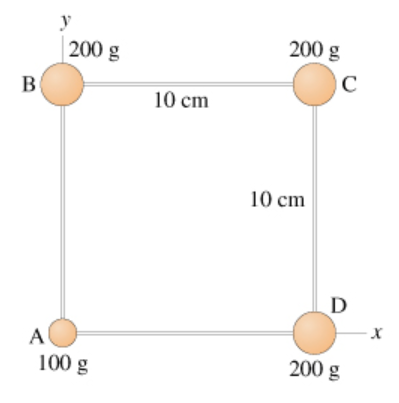# Problem: The four masses shown in the figure are connected by massless, rigid rods.a) Find the coordinates of the center of mass. b) Find the moment of inertia about an axis that passes through mass A and is perpendicular to the page.c) Find the moment of inertia about a diagonal axis that passes through masses B and D.

###### FREE Expert Solution

x-coordinate of the center of mass;

$\overline{){{\mathbf{x}}}_{\mathbf{c}\mathbf{m}}{\mathbf{=}}\frac{{\mathbf{m}}_{\mathbf{1}}{\mathbf{x}}_{\mathbf{1}}\mathbf{+}{\mathbf{m}}_{\mathbf{2}}{\mathbf{x}}_{\mathbf{2}}\mathbf{+}\mathbf{.}\mathbf{.}\mathbf{.}\mathbf{+}{\mathbf{m}}_{\mathbf{n}}{\mathbf{x}}_{\mathbf{n}}}{{\mathbf{m}}_{\mathbf{1}}\mathbf{+}{\mathbf{m}}_{\mathbf{2}}\mathbf{+}\mathbf{.}\mathbf{.}\mathbf{.}\mathbf{+}{\mathbf{m}}_{\mathbf{n}}}}$

Similarly, y-coordinate of the center of mass:

$\overline{){{\mathbf{y}}}_{{\mathbf{cm}}}{\mathbf{=}}\frac{{\mathbf{m}}_{\mathbf{1}}{\mathbf{y}}_{\mathbf{1}}\mathbf{+}{\mathbf{m}}_{\mathbf{2}}{\mathbf{y}}_{\mathbf{2}}\mathbf{+}\mathbf{.}\mathbf{.}\mathbf{.}\mathbf{+}{\mathbf{m}}_{\mathbf{n}}{\mathbf{y}}_{\mathbf{n}}}{{\mathbf{m}}_{\mathbf{1}}\mathbf{+}{\mathbf{m}}_{\mathbf{2}}\mathbf{+}\mathbf{.}\mathbf{.}\mathbf{.}\mathbf{+}{\mathbf{m}}_{\mathbf{n}}}}$

Moment of inertia:

$\overline{){\mathbf{I}}{\mathbf{=}}{\mathbf{m}}{{\mathbf{r}}}^{{\mathbf{2}}}}$

86% (343 ratings)###### Problem Details

The four masses shown in the figure are connected by massless, rigid rods.a) Find the coordinates of the center of mass.

b) Find the moment of inertia about an axis that passes through mass A and is perpendicular to the page.

c) Find the moment of inertia about a diagonal axis that passes through masses B and D.